# PDEONE Example A:

Supposed one wanted to solve the following system of partial differential equations on the interval [0,1].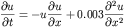This we recognized as Burgers' equation with the addition of a diffusive term. We desire the solution of the PDE above with boundary conditions given by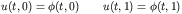here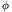is an exact solution to the PDE given by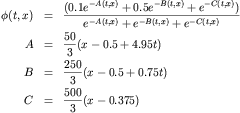With initial conditions given by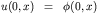This corresponds to the first example in the original reference to PDEONE (given above). This is quite simple to solve with the pdeone gateway in octave. First the user must specify an octave function "F.m" which evaluates the right hand side of the PDE expressed in the following form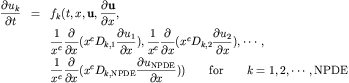The incoming arguments to the octave function F.m are t,x,u,ux, and duxx. Here t is the scalar time, x the scalar grid point, u a vector of the NPDE unknowns, ux a vector of the x derivatives of these unknowns, and duxx is a matrix expressing the following derivatives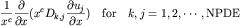With the background we can write our F.m function for evaluating the right hand side of our PDEs. In addition to F.m, PDEONE requires the information on the diffusion coefficients D. This is provided in the form of a function D.m.

PDEONE requires the boundary conditions of the form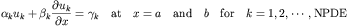To implement this in octave we must specify three boundary functions with input arguments t,x, and u (with the same meanings as above): BNDRY_ALPHA.m, BNDRY_BETA.m, and BNDRY_GAMMA.m. In the implementation BNDRY_GAMMA.m we can see one of the powers of implementing PDEs in octave. In that function we call another octave function truesol.m representing an octave implementation of the true solutionabove.

Finally the user must specify the initial conditions. In this example we can use the same function "truesol.m". The two inputs to the initial condition function are t and x. Here t is a scalar input of time but in this case x is a vector of grid points where the initial conditions are desired. Note, that to work properly this function must be vectorized meaning that it can return a matrix (of dimension [NPDE,Number of Grid Points]) of initial conditions.

Once this code is written a person can call "pdeone.m". Here we present an example pdeone_Script.m driver file. Note: Since this problem is in Cartesian the constant c=0. Since this is the default, there is no need to specify anything additional. Finally, we can reap the benefits of our work by viewing the solution to our problem: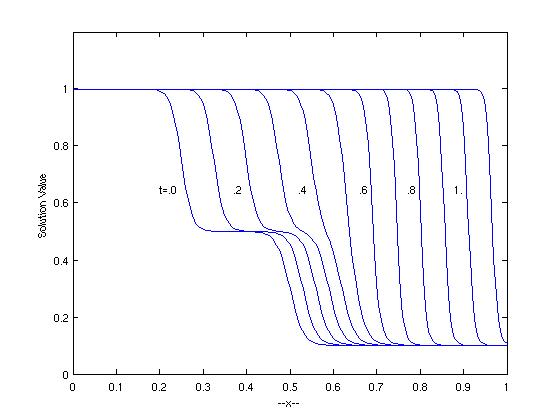Note that this is a replica of the plot given in the Sincovec and Madsen paper.
John Weatherwax